Courses
Courses for Kids
Free study material
Free LIVE classes
More

# Graph Paper with X and Y AxisLIVE
Join Vedantu’s FREE Mastercalss

## Overview of Graph With X and Y Axis

A graph paper with an x and y axis is used in mathematics and science to plot and analyse data. The x-axis and y-axis are the two perpendicular lines that intersect at the origin and divide the graph into four quadrants. These lines, also known as the coordinate axes, are used to plot points on the graph. A blank x and y-axis graph is a paper with only the axis drawn, allowing data to be plotted and analysed. The axis of a graph is the set of numerical scales used to measure and plot data on the x and y-axis.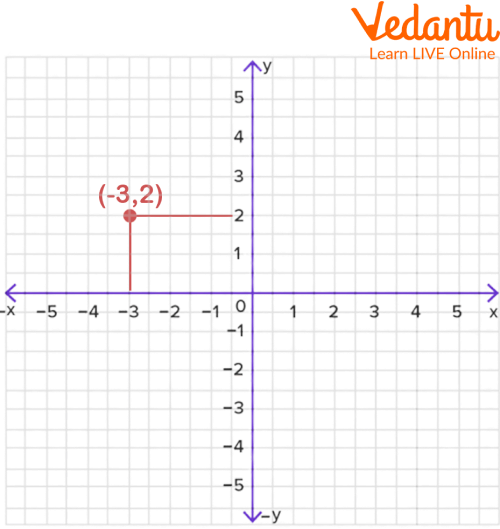Point in X Axis and Y Axis

## What is Graph?

A graph is a graphical representation or chart that represents values or data systematically. Dots on charts often represent relationships between two or more things. Charts such as graph paper with x and y axes are examples.

## What is the Axis of the Graph?

An axis on a graph is defined in mathematics as a line used to make or mark measurements. The x-axis and y-axis are the two important lines of the coordinate plane.

## X and Y Graph

The x-axis is typically horizontal, and the y-axis is typically vertical. Points can be written horizontally or vertically, so you can easily explain them using diagrams. These horizontal and vertical lines or axes on the chart are the x-axis and y-axis graphs, respectively.

An ordered pair unambiguously define every point on the coordinate plane. Ordered pairs are written as (x coordinate, y coordinate) or (x, y). where the x-coordinate is a point on the x-axis. or Perpendicular represents the distance from the y-axis, where the y-coordinate represents a point on the y-axis or perpendicular distance from the x-axis.

The X and Y axes are the axes used in the coordinate system that form the coordinate plane. The horizontal axis is represented by the x-axis, and the vertical axis by the y-axis. The following illustration shows an x ​​and y diagram with positive and negative coordinates.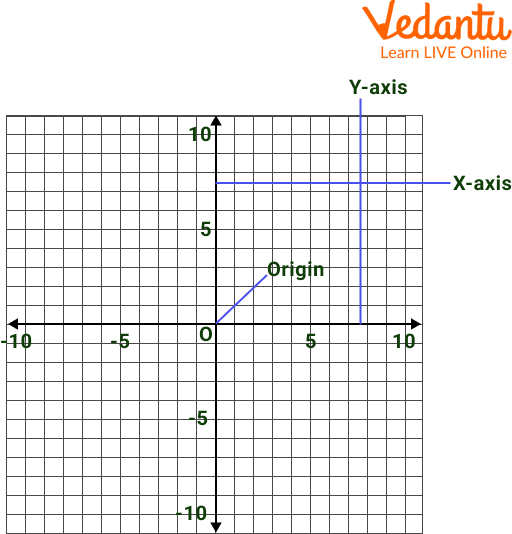X and Y Axis

## Equation of Y-Axis

The y-axis is the line whose x-coordinate value is zero for all y-values. Then the y-axis data points are (0, 4), (0, 2), (0, 1), (0, -2). So the y-axis equation is x = 0, and its plot on the x and y charts is: You can find the y coordinate in the general formula y = mx + c by replacing the x value with 0.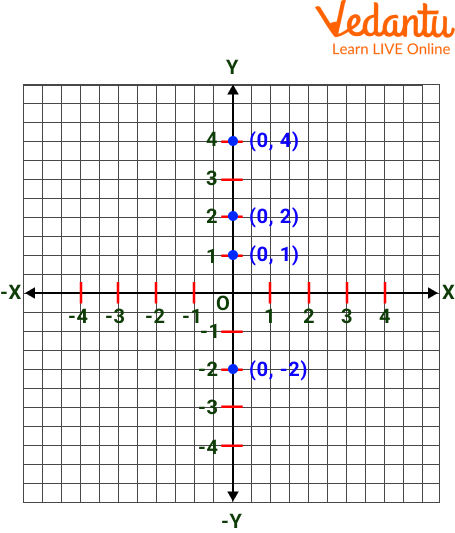Plotting of the Y-Axis

## Equation of X-axis

The x-axis is the line whose y-coordinate value is zero for all x-values. The x-axis data points are (1,0), (-2, 0). So the x-axis equation is y = 0, and its plot on the x and y graph looks like this: You can find the x coordinate by replacing the y value with 0 in the general formula y = mx + c.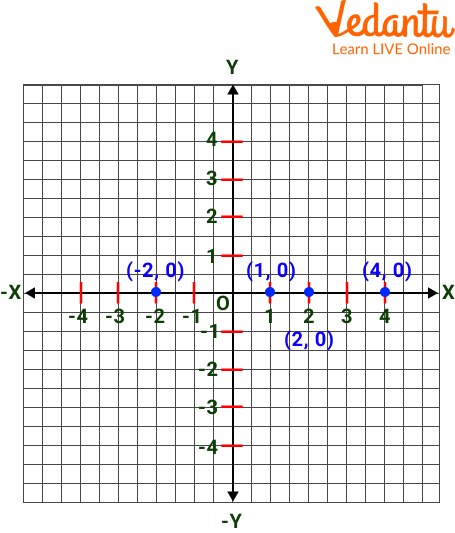Plotting of X Axis

## What is the Coordinate Plane?

Every point on the coordinate plane is unambiguously defined by an ordered pair. Ordered pairs are written as (x coordinate, y coordinate) or (x, y). where the x-coordinate is a point on the x-axis. or Perpendicular represents the distance from the y-axis, where the y-coordinate represents a point on the y-axis or perpendicular distance from the x-axis.

The X and Y axes are the axes used in the coordinate system that form the coordinate plane. The x-axis and the vertical-axis by the y-axis represent the horizontal axis. X and Y axis interaction is known as the origin and serves as the reference point for the plane. There are 4 quadrants in the graph.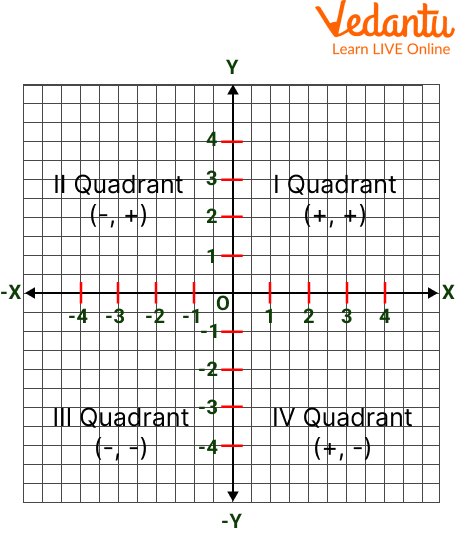## Locating a Point on the Coordinate Plane

Now that we understand coordinate planes and their parts, let's discuss how to locate points on coordinate planes. To find a point on the coordinate plane:

Step 1: Find a point.

Step 2: Find the quadrant by looking at the signs of the X and Y coordinates.

Step 3: Read the number of units the point is to the right/left of the origin along the X axis to find the X of the point.

Step 4: Find the y of the point by reading the number of units the point is above/below the origin along the y-axis.

To find a point on the coordinate plane, see the diagram and steps below.

Step 1: Observe the blue dot on the coordinate map.

Step 2: Located in the second quadrant.

Step 3: The point is 3 units from the origin along the negative X axis.

Step 4: The point is 2 units from the origin along the positive Y axis.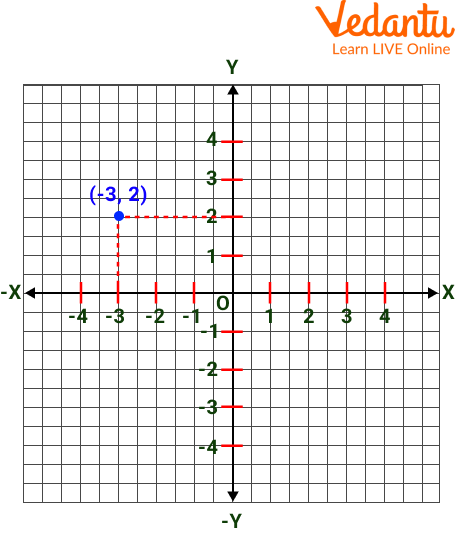Intersection Point of the X and Y Axis

## Plotting Points on a Coordinate Plane

In this section, you will learn how to draw points on the coordinate plane. Let's take the example of the point P = (4, 3). To draw a point on the coordinate plane:

Step 1: Draw two perpendicular lines for the X and Y axes.

Step 2: Start from the origin. Move 4 units to the right along the Positive(+ve) X axis.

Step 3: Move up 3 units along the positive Y axis.

Step 4: Mark the intersection. Mark as (4, 3).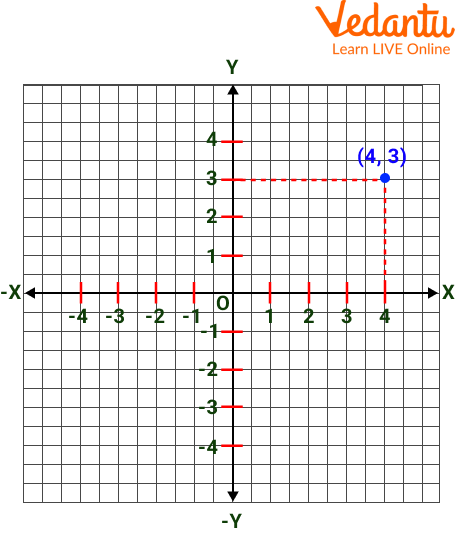Intersection Point of X and Y Axis

## Solved Question

1. Plot X= 4 Y= 2 in the first quadrant.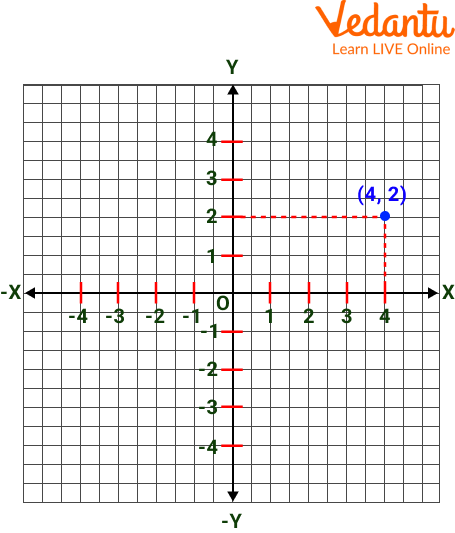Solved Question

## 2. Plot the point on graph: P(3, -2)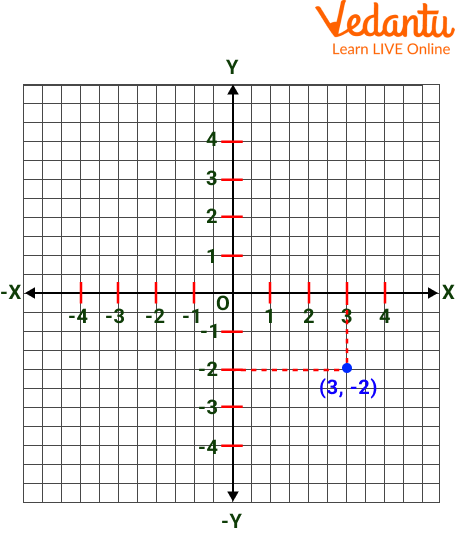Solved Question

## Practice Question

1. Plot X= +2 Y= -2

2. Plot X= -2 Y= +4

1.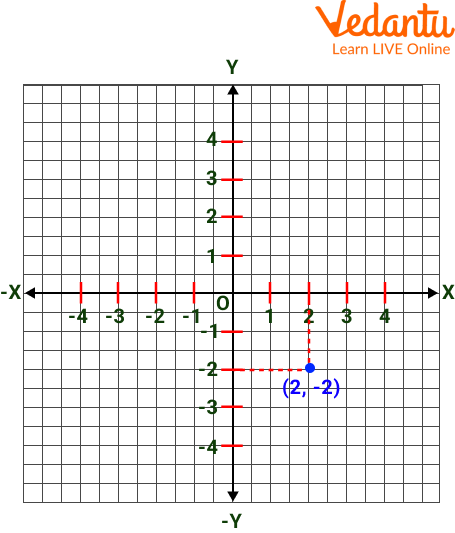Practice Question

2.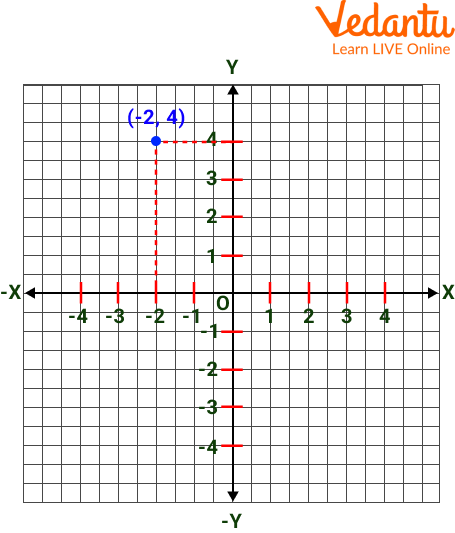Practice Question

## Summary

In this article, we have learned about graphs, and we also read about the axis of the graph, y-graph and x-graph, also known as the y-axis and x-axis are the two important lines that make up a graph. A graph consists of horizontal and vertical axes on which data can be plotted. And also learned about the coordinate plane, a two-dimensional plane formed by the intersection of the x-axis (horizontal line) and the y-axis (vertical line). We also solved some examples on this and some questions for practice will help you to solve quickly.

Last updated date: 26th Sep 2023
Total views: 70.5k
Views today: 2.70k

## FAQs on Graph Paper with X and Y Axis

1. What did we call the x-axis?

It is known as the abscissa axis.

2. What did we call the y-axis?

It is known as the Ordinate-axis.

3. What are the types of lines in a coordinate plane?

The types of lines in a coordinate plane are parallel lines, intersecting lines, Skew Lines, coplanar lines, and coincident lines. All these lines can be drawn with reference to the x, y, and z-axis in the coordinate plane.